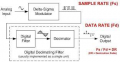# What is the difference between ADC sampling rate and data rate

#### hoyyoth

Joined Mar 21, 2020
289
Dear Team,

May I know the difference between ADC sampling rate and data rate .

If the sampling rate is 125Ksps,can I say data rate is also 125Ksps

#### hoyyoth

Joined Mar 21, 2020
289
That is a general explanation.if you don't mind can you give an answer more specific to ADC

#### Jerry-Hat-Trick

Joined Aug 31, 2022
450
My understanding is that ADC (Analog to Digital Conversion) sampling rate is the number of samples per second, whereas data rate usually refers to the number of bit transferred in one second. So if you are sampling an analog signal with 10 bit resolution (i.e. 2^10 = 1024 so about 0.1% of the scale per bit) 1,000 times a second you'd need to pass this through a serial link at a data rate of 10,000 bits per second, plus maybe a bit of overhead.

#### WBahn

Joined Mar 31, 2012
29,510
Dear Team,

May I know the difference between ADC sampling rate and data rate .

If the sampling rate is 125Ksps,can I say data rate is also 125Ksps
Depends on what data you are talking about. Usually data rate is expressed in either bits/sec or bytes/sec, not in samples/second.

#### drjohsmith

Joined Dec 13, 2021
816
Yes
Dear Team,

May I know the difference between ADC sampling rate and data rate .

If the sampling rate is 125Ksps,can I say data rate is also 125Ksps
Yes you can.
If your sampling at 125 ksps then yiu have 125000 samples per second.
Assuming the adc is putting out in digitally all the samples that is. Most do.
The data rate is the number of bits in each sample times the sample rate
So if 10 bits, then that would be 1250 kbit per second , or another way 1.25 Mbit per second,
But it's still 125k samples per second.

•hoyyoth

#### hoyyoth

Joined Mar 21, 2020
289
Thank you.
That explanation is very clear.

•drjohsmith

#### nsaspook

Joined Aug 27, 2009
12,298
Thank you.
That explanation is very clear.
It depends on the the type of ADC. For a delta-sigma ADC you have the sample-rate (that might be in the MHz), the Decimation 'rate/ratio' and a data rate for the converted digital bits.Although most converters have only one sample rate, delta-sigma converters have two: the input sampling rate and the output-data rate. The ratio of these two meaningful variables defines the system’s decimation ratio. A strong relationship exists between the decimation ratio and the converter’s effective resolution.

•hoyyoth

#### drjohsmith

Joined Dec 13, 2021
816
It depends on the the type of ADC. For a delta-sigma ADC you have the sample-rate (that might be in the MHz), the Decimation 'rate/ratio' and a data rate for the converted digital bits.
View attachment 303050